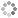# PROWAREtecharticles » current » javascript » tutorial » page-09

## JavaScript: Tutorial - A Guide to JavaScript - Page 09

Language Basics (Functions, Reference Types: The Object Type).

## Functions

A function is declared using the `function` keyword, followed by a set of arguments and then the body of the function.

``````function square(n) {
return n * n;
}
function showMessage(message) {
}
showMessage("Hello, World!"); // shows "Hello, World!"
var num = square(12);		 // num is 144
showMessage(square(9));		 // shows "81"``````

#### Functions as Values

Functions can be used any place any other value can be used because function names are variables. This means it is possible to pass a function into another function as an argument and also to return a function as the result of another function.

``````function callSomeFunction(someFunction, someArgument){
return someFunction(someArgument);
}
function square(num){
return num * num;
}
var result = callSomeFunction(square, 8);
``````function createComparisonFunction(propertyName){
return function(object1, object2){
var value1 = object1[propertyName];
var value2 = object2[propertyName];
if (value1 < value2){
return -1;
}
else if (value1 > value2){
return 1;
}
else{
return 0;
}
};
}``````

#### Recursion

Recursion happens when a function calls itself. `arguments.callee` is a pointer to the function executing.

``````function f(num){
if (num <= 1){
return 1;
} else {
return num * arguments.callee(num-1); // arguments.callee is the f function.
}										 // return num * f(num-1); is equivalent.
}``````

The arguments object acts like an array. One can specify as many arguments as one wishes. Access each argument using bracket notation. As below:

``````var globalVar = 0;						// globalVar is a global variable
function sum() {
var retval = 0;					 // retval is a private variable
for(var i = 0; i < arguments.length; i++) {
retval += arguments[i];
}
return retval;
}
var sum1 = sum(1, 3, 5, 7, 10, 11, 15); // sum1 == 52
var sum2 = sum(10, 12, 15);			 // sum2 == 37

## Reference Types

### The Object Type

There are two ways to create an object. First, use the `new` operator with the `Object` constructor.

``````var animal = new Object();
animal.type = "dog";
animal["name"] = "Napolean";

Objects created with the Object constructor, as above, are always passed by reference instead by value.

Second, use object literal notation.

``````var animal = {
type: "dog",
name: "Napolean"
};

Or:

``````var animal = {}
animal.type = "dog";
animal.name = "Napolean";

There are several different ways to access `Object` properties.

``````var animal = {
type: "dog",
name: "Napolean"
};
var propertyName = "name";

Be careful when working with the `Object` type. It is assigned by reference only. In otherwords, the variable is actually a pointer to the object.

``````var person = new Object();
person.name = "Joe Bleaux";
person.age = 93;

var oldman = person;This site uses cookies. Cookies are simple text files stored on the user's computer. They are used for adding features and security to this site. Read the privacy policy.